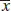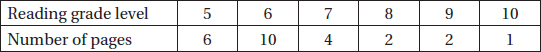# AP Statistics Multiple-Choice Practice Questions: Tests of Significance-Proportions and Means 2

### Test Information14 questions32 minutes

1. A pharmaceutical company claims that 8% or fewer of the patients taking their new statin drug will have a heart attack in a 5-year period. In a government-sponsored study of 2300 patients taking the new drug, 198 have heart attacks in a 5-year period. Is this strong evidence against the company claim?

2. Is Internet usage different in the Middle East and Latin America? In a random sample of 500 adults in the Middle East, 151 claimed to be regular Internet users, while in a random sample of 1000 adults in Latin America, 345 claimed to be regular users. What is the P-value for the appropriate hypothesis test?

3. What is the probability of mistakenly failing to reject a false null hypothesis when a hypothesis test is being conducted at the 5% significance level (α = 0.05)?

4. A research dermatologist believes that cancers of the head and neck will occur most often of the left side, the side next to a window when a person is driving. In a review of 565 cases of head/neck cancers, 305 occurred on the left side. What is the resulting P-value?

5. Suppose you do five independent tests of the form H0: µ = 38 versus Ha: µ > 38, each at the α = 0.01 significance level. What is the probability of committing a Type I error and incorrectly rejecting a true null hypothesis with at least one of the five tests?

6. Given an experiment with H0: µ = 35, Ha: µ < 35, and a possible correct value of 32, you obtain a sample statistic of= 33. After doing analysis, you realize that the sample size n is actually larger than you first thought. Which of the following results from reworking with the increase in sample size?

7. Thirty students volunteer to test which of two strategies for taking multiple-choice exams leads to higher average results. Each student flips a coin, and if heads, uses Strategy A on the first exam and then Strategy B on the second, while if tails, uses Strategy B first and then Strategy A. The average of all 30 Strategy A results is then compared to the average of all 30 Strategy B results. What is the conclusion at the 5% significance level if a two-sample hypothesis test, H0: µ1 = µ2, Ha: µ1µ2,results in a P-value of 0.18?

8. Choosing a smaller level of significance, that is, a smaller α-risk, results in

9. The greater the difference between the null hypothesis claim and the true value of the population parameter,

10. A company selling home appliances claims that the accompanying instruction guides are written at a 6th grade reading level. An English teacher believes that the true figure is higher and with the help of an AP Statistics student runs a hypothesis test. The student randomly picks one page from each of 25 of the company's instruction guides, and the teacher subjects the pages to a standard readability test. The reading levels of the 25 pages are given in the following table:Assuming that the conditions for inference are met, is there statistical evidence to support the English teacher's belief?

11. Suppose H0: p = 0.4, and the power of the test for the alternative hypothesis p = 0.35 is 0.75. Which of the following is a valid conclusion?

12. A factory is located close to a city high school. The manager claims that the plant's smokestacks spew forth an average of no more than 350 pounds of pollution per day. As an AP Statistics project, the class plans a one-sided hypothesis test with a critical value of 375 pounds. Suppose the standard deviation in daily pollution poundage is known to be 150 pounds and the true mean is 385 pounds. If the sample size is 100 days, what is the probability that the class will mistakenly fail to reject the factory manager's false claim?

13. For which of the following is a matched pairs t-test not appropriate?

14. Do high school girls apply to more colleges than high school boys? A two-sample t-test of the hypotheses H0: µgirls = µboys versus Ha: µgirls > µboys results in a P-value of 0.02.

Which of the following statements must be true?

I.A 90% confidence interval for the difference in means contains 0.

II.A 95% confidence interval for the difference in means contains 0.

III.A 99% confidence interval for the difference in means contains 0.